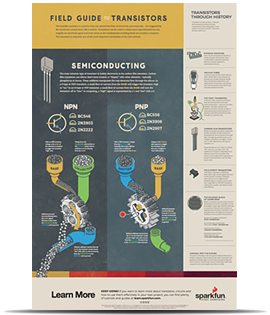# Transistors

Pages
Contributors:jimblom

## Introduction

Transistors make our electronics world go 'round. They're critical as a control source in just about every modern circuit. Sometimes you see them, but more-often-than-not they're hidden deep within the die of an integrated circuit. In this tutorial we'll introduce you to the basics of the most common transistor around: the bi-polar junction transistor (BJT).

In small, discrete quantities, transistors can be used to create simple electronic switches, digital logic, and signal amplifying circuits. In quantities of thousands, millions, and even billions, transistors are interconnected and embedded into tiny chips to create computer memories, microprocessors, and other complex ICs.

### Covered In This Tutorial

After reading through this tutorial, we want you to have a broad understanding of how transistors work. We won't dig too deeply into semiconductor physics or equivalent models, but we'll get deep enough into the subject that you'll understand how a transistor can be used as either a switch or amplifier.

This tutorial is split into a series of sections, covering:

There are two types of basic transistor out there: bi-polar junction (BJT) and metal-oxide field-effect (MOSFET). In this tutorial we'll focus on the BJT, because it's slightly easier to understand. Digging even deeper into transistor types, there are actually two versions of the BJT: NPN and PNP. We'll turn our focus even sharper by limiting our early discussion to the NPN. By narrowing our focus down -- getting a solid understanding of the NPN -- it'll be easier to understand the PNP (or MOSFETS, even) by comparing how it differs from the NPN.Before digging into this tutorial, we'd highly recommend giving these tutorials a look-through:

• Voltage, Current, Resistance, and Ohm's Law -- An introduction to the fundamentals of electronics.
• Electricity Basics -- We'll talk a bit about electricity as the flow of electrons. Find out how those electrons flow in this tutorial.
• Electric Power -- One of the transistors main applications is amplifying -- increasing the power of a signal. Increasing power means we can increase either current or voltage, find out why in this tutorial.
• Diodes -- A transistor is a semiconductor device, just like a diode. In a way, it's what you'd get if you stacked two diodes together, and tied their anodes together. Understanding how a diode works will go a long way towards uncovering the operation of a transistor.

## Looking to explore transistors?

We've got you covered!

KIT-13973
\$18.50
12

KIT-13682
\$12.95
6

COM-00521
\$0.55

COM-13689
\$0.55